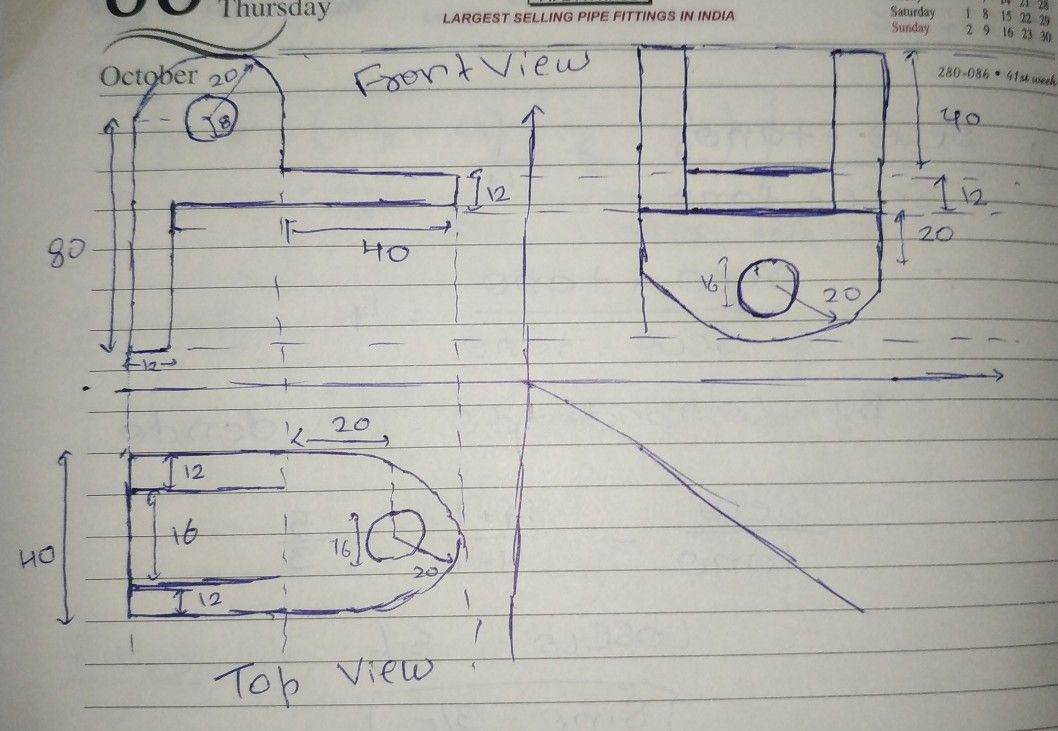Symbol
Problem$87$ $→$ $22$ $4$ $→$ $s$ 12.16.12. $-7$ $98$ $\vec{\infty }$ $2$ 6 1$<2$ $y$
Other
Question content
draw front view top view and both side view of given figure when it is looking from given X direction. sir you may draw rough digram with proper dimensions specially at critical curved positions.
SolutionQanda teacher - Atharvaif you want printed solution then I may arrenge it for you
if you wish
thank you
best of luckStudent
thank you sirQanda teacher - Atharva
please rate